# 10 Best and Free Maths Apps for Android | 2020

Mathematics is one of the most hated subjects by students. We all know, it is one of the hardest subjects that we learn in our schools. Whether you are young or grown-ups, the Math haunts everyone. Some people feel more nervous about maths or related exams than any other subject. If you feel the same and want to fight with your fear then these Mathematics apps can help you in achieving your goal.

These maths apps help you to fight your maths fear in a new way. You will never feel nervous in maths and become confident and fast in solving maths problems by using these free mathematics apps. Also, soon you will be the master in maths subject with the help of these apps.

## Best and Free Maths Apps

Below is the list of best and free maths apps to use for your maths-related problems. You can use any of them as per your need.

### 1. Microsoft Math Solver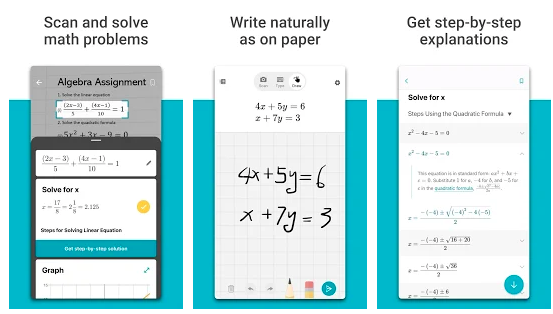The Microsoft Math solver app is one of the best free mathematics apps that help with a variety of problems including arithmetic, algebra, trigonometry, calculus, statistics, and other topics. The app uses an advanced AI-powered math solver. You just have to write a problem on the screen or use the camera to snap a math photo. After that Microsoft Math problem solver app instantly recognizes the problem and helps you in solving it with Step-By-Step Explanations.

### 2. Maths Tricks: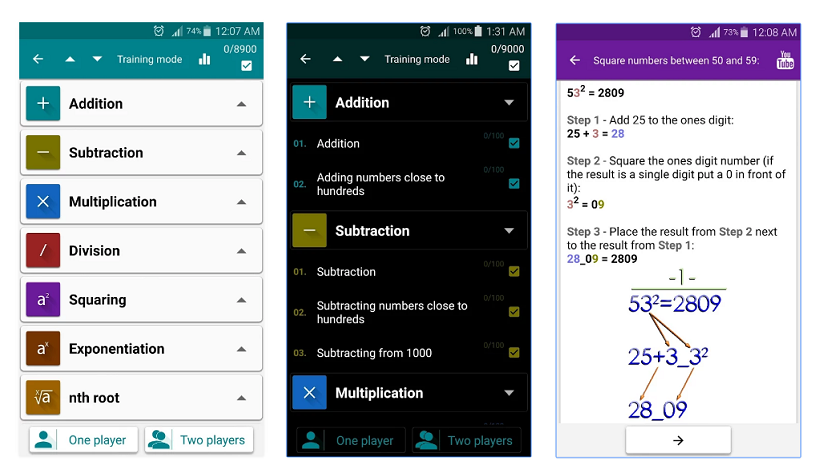The Maths Tricks app is for those people who are maths lovers and want to learn interesting mathematical tricks and shortcuts to speed up the calculating. Tricks mentioned in this app will help in solving part of the mathematical problems and help in doing tasks much faster. It also has basics like the multiplication table and advances like n^9.

The quick calculation by this app helps in saving a lot of precious time. It also has a friends list where you are able to show off your skills to friends. This will be fun as you can prove to them that you are the great mathematician.

### 3. Photomath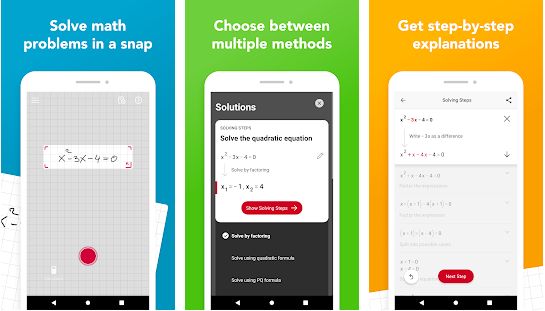With the Photomath maths learning app, you can learn math, check homework and study for upcoming tests and ACTs/SATs. This app can solve tricky homework or class assignments with step-by-step explanations and animations. The best point to be noted that it is free and works without wi-fi or data. So, whether you’re on the bus, or just offline, core Photomath is always available.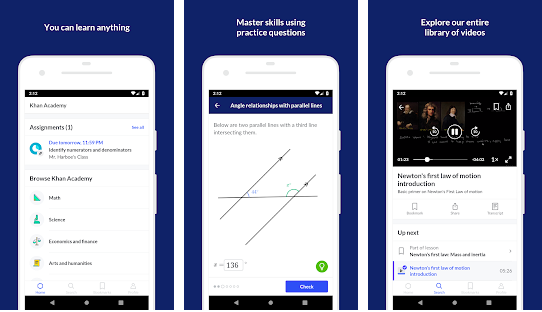Khan Academy is one of the educational apps that have many subjects and math is one of them. This math app has in-depth articles in math with problems based on arithmetic, pre-algebra, algebra, geometry, trigonometry, statistics, calculus, linear algebra. The app includes over 10,000 videos, 40,000 questions, and tons of different types of math.

### 5. Maths expert: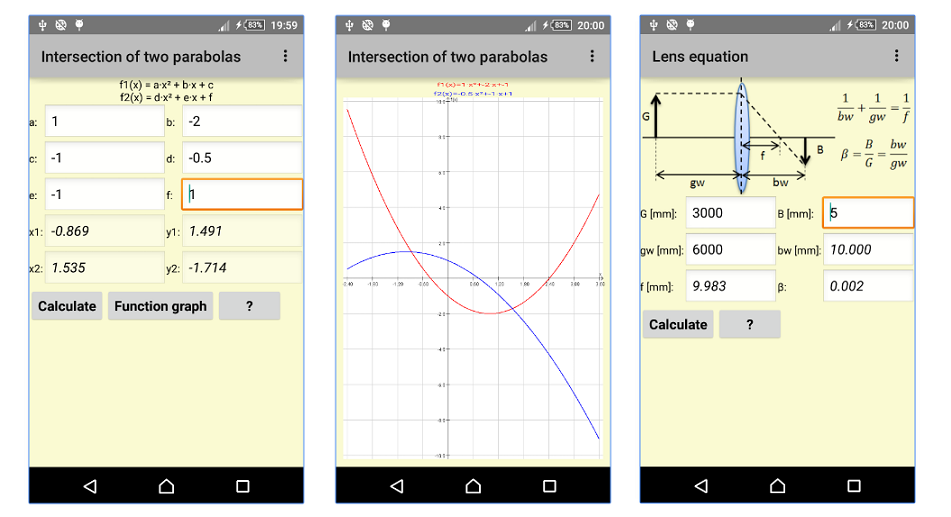This maths app is really an expert in maths field. It can calculate from simple formulas to parabolas. Math expert is a collection of all formulas from mathematics and physics. The calculation is based on the motto “Tell me what you know, and I will check which calculations are possible.”You will definitely feel awesome after using this Maths expert and I think this is the must-have apps if you are in the field of maths and science.

You will definitely feel awesome after using this Maths expert and I think this is the must-have apps if you are in the field of maths and science.

Also read: Best offline dictionary Apps

### 6. Photomath- Camera Calculator: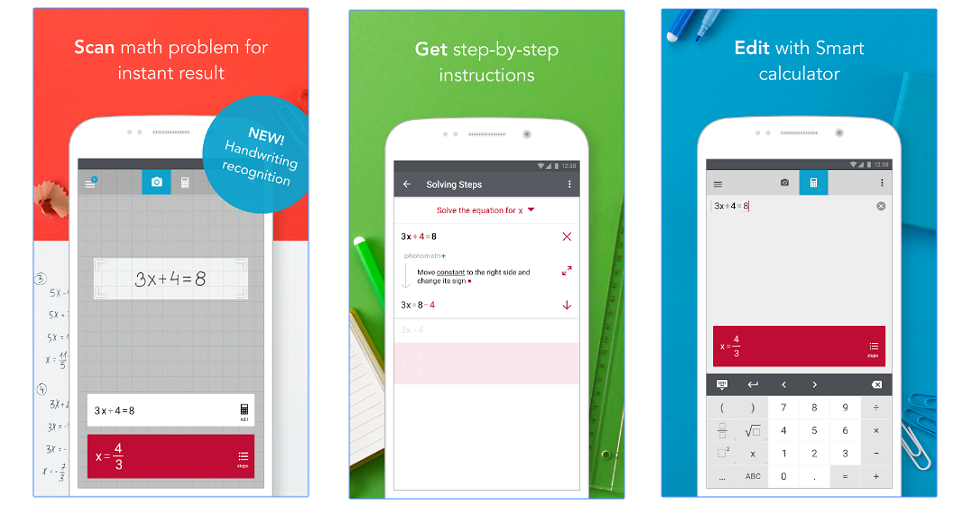Photomath provides Camera calculator, Handwriting recognition (NEW), Step-by-step instructions and Smart calculator which gives a perfect camera calculator. Just you have to simply point your camera toward a math problem and this maths app will magically show the result. And that too with detailed step-by-step instructions.

### 7. Maths Dictionary Offline: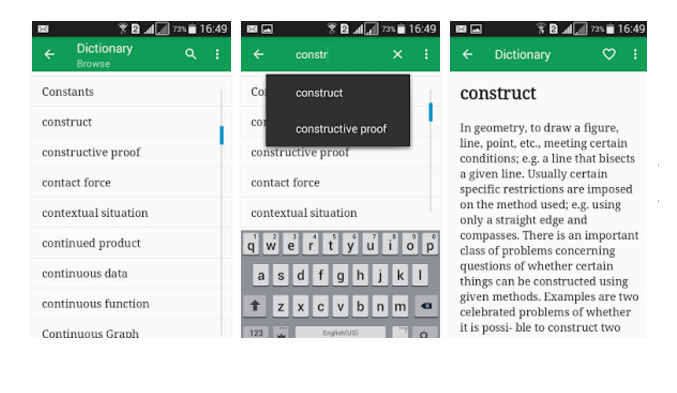Math Dictionary Offline is a learning and search tool which has an Alphabetical listing of all mathematical terms. you do not need an internet connection as this mathematics app works offline. So, you can get help and trips when no data connection is available.
There are thousands of Math Words and Terms in this mathematics apps and it is completely free. No need to pay anything ever.

### 8. Maths Formula Free:You can calculate any formula related to Geometry, Algebra, Trigonometry, Equations, Analytic Geometry, Differentiation, Integration, Matrix, Probability, and statistics. A good feature of this app is that it is available in many languages. It’s very easy to use by all students and engineers to look for any easy or complicated formulas.
This maths app also calculates the geometric shapes or find the roots of equations. You can easily share any formulas with your friends by email, message, or Facebook with the help of this app.

### 9. GMAT Math Flashcards: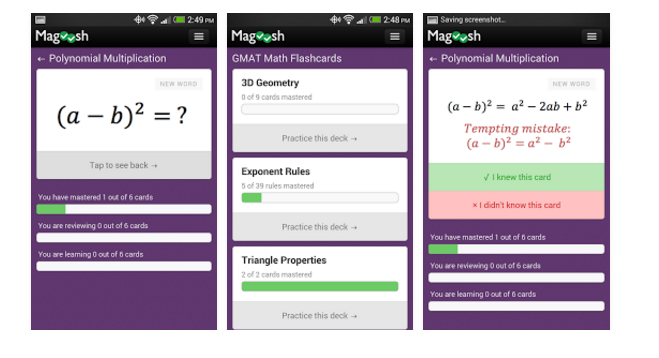This app is made by Magoosh. You can learn GMAT math formulas with the help of this maths app and can solve GMAT questions with free flashcards. You can practice daily which can improve your score in GMAT. Also, there is a privilege in this app that you are free to access all of Magoosh’s GMAT math flash cards.

### 10. MathsApp – Vedic Math Tricks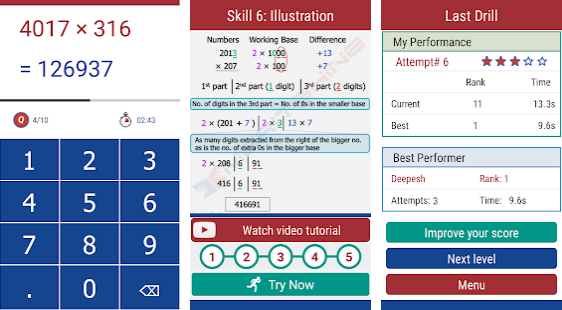This Vedic maths app is tricks and speed calculation app which helps you in learning and practicing various interesting speed calculation and math tricks. You can learn math skills to save time with the help of this app which can use at school, college, or at work.